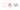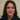# Javascript useful every() method explained with examples

Following previous article around Javascript methods we cover every() method in this article as one of the important ones.

## The every() method

Just like some() method every() method also needs to check if a condition is true within elements of an array.

However the difference is it tests whether all elements in the array pass the test implemented by the provided function. We will get `true` for any condition put on an empty array too.

Basically if it finds a falsy value that does not meet the condition it immediately returns false. We better take a look at some examples.

### A simple example

As an example we could check whether a size is small enough or not:

``````function isSmallEnough(element, index, array) {
return element <= 10;
}<br/>// Checking two arrays for the condition
[12, 5, 8, 130, 44].every(isSmallEnough); // false
[2, 5, 0, 10, 6].every(isSmallEnough); // true``````

We see that some values of the first array does not meet the condition. Therefor we get a false result while in the second one it passes the condition for all item values.

### Testing objects for a value

Generally we can also test every() method on objects to see if they pass the test. Have a look at this example:

``````const arr1 = [{a:1, b:2, c:3, d:4}, {a:1, x:2, y:3, z:4}, {a:1, x:2, y:3, z:4}];<br/>arr1.every(obj => obj.a === 1); //true

const arr2 = [{a:1, b:2, c:3, d:4}, {a:1, x:2, y:3, z:4}, {a:2, x:2, y:3, z:4}];<br/>arr2.every(obj => obj.a === 1); //false``````

We see in the second object only one pair does not meet the condition. As a result every() method returns false no matter the rest of pairs pass the condition.

Moreover we can use arrow function in this method as well. Check out these examples:

``````const a1 = [12, 5, 8, 130, 44];<br/>a1.every(x => x >= 10); // false<br/><br/>// Or all in one line
[12, 54, 18, 130, 44].every(x => x >= 10); // true``````

Generally we need to use every() method once we need a condition to be met in every single array item. Therefor it is like an && operator in practice and should be used in appropriate places. Thanks for reading.